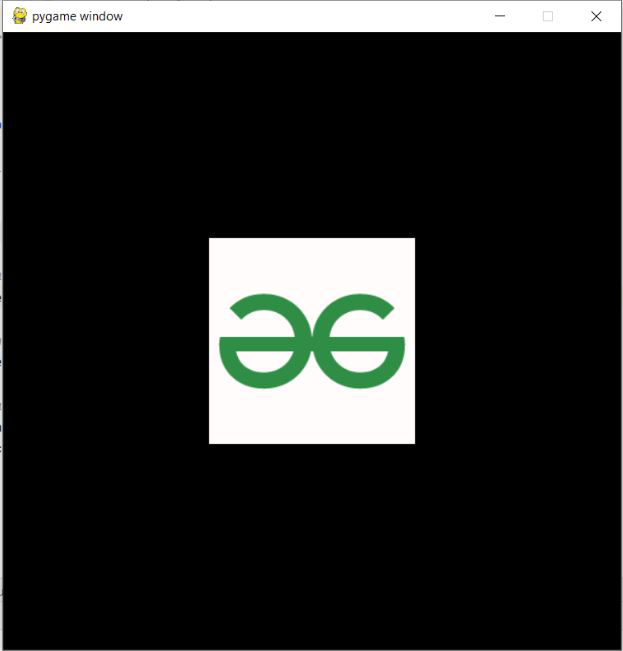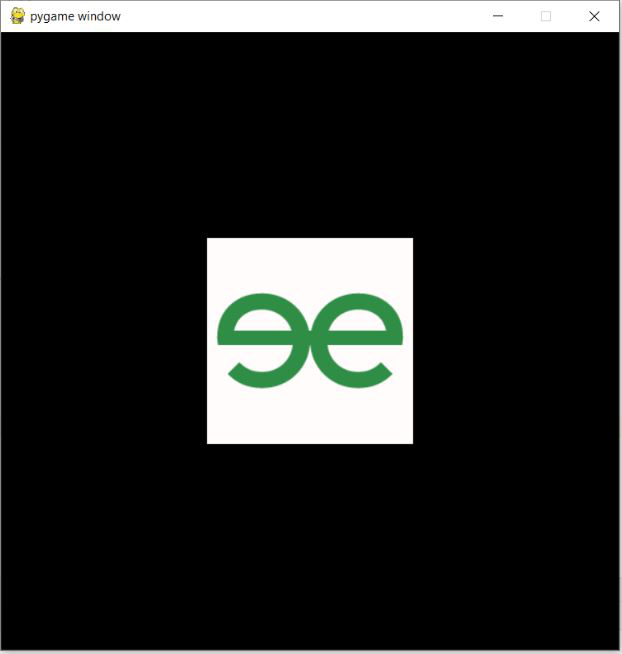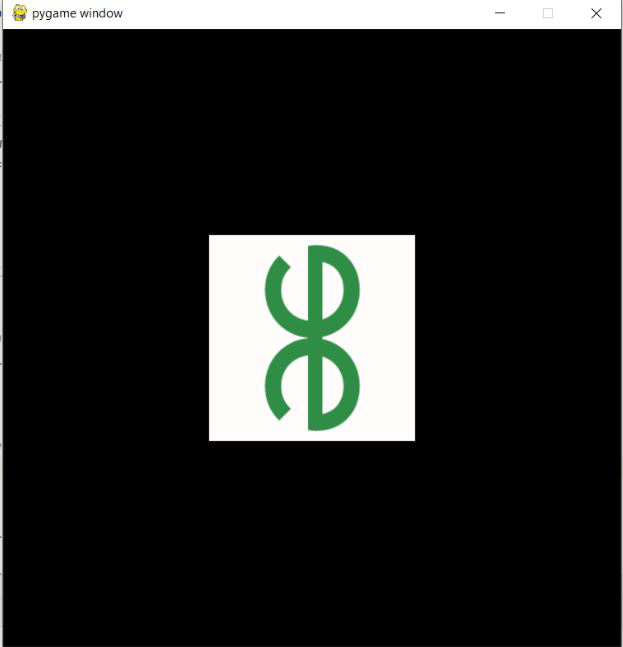GeeksforGeeks App
Open AppBrowser
Continue

# How to Rotate and Scale images using PyGame ?

In this article, we are going to see how to Rotate and Scale the image. Image Scaling refers to the resizing of the original image and Image Rotation refers to turning off an image with some angle. Rotations in the coordinate plane are counterclockwise. Let’s proceed with the methods used and the complete code to perform these tasks.

## Scaling the Image

To scale the image we use the pygame.transform.scale(image, DEFAULT_IMAGE_SIZE) method where we pass the image that we are going to scale and the default image size that we will set manually according to our need.

Example:

Image Used:## Python3

 `# Import pygame``import` `pygame` `# Initialise pygame``pygame.init()` `# Set window size``size ``=` `width,height ``=` `600``, ``600``screen ``=` `pygame.display.set_mode(size)` `# Clock``clock ``=` `pygame.time.Clock()` `# Load image``image ``=` `pygame.image.load(``'gfg.png'``)` `# Set the size for the image``DEFAULT_IMAGE_SIZE ``=` `(``200``, ``200``)` `# Scale the image to your needed size``image ``=` `pygame.transform.scale(image, DEFAULT_IMAGE_SIZE)` `# Set a default position``DEFAULT_IMAGE_POSITION ``=` `(``200``,``200``)` `# Prepare loop condition``running ``=` `False` `# Event loop``while` `not` `running:` `    ``# Close window event``    ``for` `event ``in` `pygame.event.get():``        ``if` `event.``type` `=``=` `pygame.QUIT:``            ``running ``=` `True` `    ``# Background Color``    ``screen.fill((``0``, ``0``, ``0``))` `    ``# Show the image``    ``screen.blit(image, DEFAULT_IMAGE_POSITION)` `    ``# Part of event loop``    ``pygame.display.flip()``    ``clock.tick(``30``)`

Output:## Rotating the Image

To rotate the image we use the pygame.transform.rotate(image, degree) method where we pass the image that we are going to rotate and the degree by which rotation is to be done.

Example:

## Python3

 `# Import pygame``import` `pygame` `# Initialise pygame``pygame.init()` `# Set window size``size ``=` `width,height ``=` `600``, ``600``screen ``=` `pygame.display.set_mode(size)` `# Clock``clock ``=` `pygame.time.Clock()` `# Load image``image ``=` `pygame.image.load(``'gfg.png'``)` `# Set the size for the image``DEFAULT_IMAGE_SIZE ``=` `(``200``, ``200``)` `# Rotate the image by any degree``image ``=` `pygame.transform.rotate(image, ``180``)` `# Set a default position``DEFAULT_IMAGE_POSITION ``=` `(``200``,``200``)` `# Prepare loop condition``running ``=` `False` `# Event loop``while` `not` `running:` `    ``# Close window event``    ``for` `event ``in` `pygame.event.get():``        ``if` `event.``type` `=``=` `pygame.QUIT:``            ``running ``=` `True` `    ``# Background Color``    ``screen.fill((``0``, ``0``, ``0``))` `    ``# Show the image``    ``screen.blit(image, DEFAULT_IMAGE_POSITION)` `    ``# Part of event loop``    ``pygame.display.flip()``    ``clock.tick(``30``)`

Output:## Rotating and Scaling the Image

Let us see how to perform the Scaling and Rotation of an image given.  We will set the default image size that is agreeable and the default image position where we want to see our image on the window screen. The same methods that are explained above will be used for scaling and rotation the image.

Example:

## Python3

 `# Import pygame``import` `pygame` `# Initialise pygame``pygame.init()` `# Set window size``size ``=` `width,height ``=` `600``, ``600``screen ``=` `pygame.display.set_mode(size)` `# Clock``clock ``=` `pygame.time.Clock()` `# Load image``image ``=` `pygame.image.load(``'gfg.png'``)` `# Set the size for the image``DEFAULT_IMAGE_SIZE ``=` `(``200``, ``200``)` `# Scale the image to your needed size``image ``=` `pygame.transform.scale(image, DEFAULT_IMAGE_SIZE)` `# Rotate the image by any degree``image ``=` `pygame.transform.rotate(image, ``90``)` `# Set a default position``DEFAULT_IMAGE_POSITION ``=` `(``200``,``200``)` `# Prepare loop condition``running ``=` `False` `# Event loop``while` `not` `running:` `    ``# Close window event``    ``for` `event ``in` `pygame.event.get():``        ``if` `event.``type` `=``=` `pygame.QUIT:``            ``running ``=` `True` `    ``# Background Color``    ``screen.fill((``0``, ``0``, ``0``))` `    ``# Show the image``    ``screen.blit(image, DEFAULT_IMAGE_POSITION)` `    ``# Part of event loop``    ``pygame.display.flip()``    ``clock.tick(``30``)`

Output:My Personal Notes arrow_drop_up